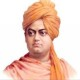# Mixed Quantitative Aptitude Questions Set 55

Quantitative Aptitude Questions for IBPS RRB/PO/Clerk, SBI PO, NIACL, NICL, RBI Grade B/Assistant, BOI, Bank of Baroda and other competitive exams

1. A profit of 30% is made on goods when a discount of 20% is given on the marked price. What profit per cent will be made when a discount of 30% is given on the marked price?
A) 11
B) 13.75
C) 12.5
D) 6.5
E) None
Option B
Solution:

discount 80 130 (profit)
MP 100 ? ==> (130*5)/4
Then 100 (130*5)/4
Discount 70 ? ==> (13*5*7)/4
=113.75.
2. A shopkeeper marks up the price of his product by 20%. If he increases the discount from 5% to 10% ,the proﬁt would decrease by Rs 21. How much proﬁt/ loss would he earn if he gives a discount of 20% on the marked price?
A) Rs14 loss
B) Rs14 profit
C) Rs20 loss
D) Rs20 profit
E) None
Option A
Solution:

Let CP be 100 then MP 120
5% discount =114.
10% discount =108.
Diff 6 21
(CP)100 ?==> 350.
Then MP=20% of 350=70=350+70=420.
Now 20% discount 20% of 420 =336.
Loss =350-336=Rs14.
3. A number, x equals 80% of the average of 5, 7, 14 and a number y. If the average of x and y is 26, then value of y is
A) 28
B) 36
C) 25
D) 39
E) None
Option D
Solution:

Average of 5,7,14 and y=(5+7+14+y) /4
Then x= 80% of (5+7+14+y) /4
x=(26+y) /5–1
(x+y)/2=26 –2
Solving 1 and 2 y=39.
4. The average age of a family of 6 members is 20 years. If the age of the youngest member be 5 years, the average age of the family at the birth of the youngest member was?
A) 19 yrs
B) 22 yrs
C) 16 yrs
D) 21 yrs
E) None
Option A
Solution:

Total present age of the family (6*20) = 120 yrs
Total age of the family 6 years ago = (120 – 6*5) = 90 years
At that time, Total members in the family = 5
Therefore Average age at that time = 90/5 = 19 yrs..
5. The distance between two cities A and B is 330km. A train starts from A at 7am. and travels towards B at 60 km/hr. Another train starts from B at 8am. and travels towards A at 75 km/hr. At what time do they meet?
A) 11am
B) 11.30am
C) 10.30am
D) 10am
E) None
Option D
Solution:

Distance travelled by first train in one hour
= 60 x 1 = 60 km
Therefore, distance between two train at 9 a.m.
= 330 – 60 = 270 km
Now, Relative speed of two trains = 60 + 75 = 135 km/hr
Time of meeting of two trains =270/135=2 hrs.
Therefore, both the trains will meet at 9 + 2 = 10 A.M.
6. Speed of a man in still water is 6 km/hr and the river is running at 4km/hr. The total time taken to go to a place and come back is 18 hours. What is the distance traveled?
A) 45km
B) 40km
C) 60km
D) 50km
E) None
Option C
Solution:

Down speed= 6+4= 10
Up speed= 6-4=2
Let distance travelled = X
(X/10)+(X/2)= 18
X= 30 km
Total distance is 30+30=60.
7. A tricolor flag is to be formed having three adjacent strips of three different colors chosen from six different colors. How many different colored flags can be formed with different design in which all the three strips are always in horizontal positions?
A) 110
B) 90
C) 120
D) 85
E) None
Option C
Solution:

First strips can be coloured in 6ways and second strip can be coloured in 5ways and third strip can be coloured in 4ways.
Hence all the strips can be coloured in 6*5*4=120ways.
8. There are 7 men and 8 women. In how many ways a committee of 4 members can be made such that a particular woman is always included.
A) 380
B) 410
C) 290
D) 364
E) None
Option D
Solution:

There are total 15 people, a particular woman is to be included, so now 14 people are left to chosen from and 3 members to be chosen.
So ways are 14C3=(14*13*12) /(3*2*1)
=364.
9. Fresh fruit contains 68% water and dry fruit contains 20% water. How much dry fruit can be obtained from 100 kg of fresh fruits ?
A) 40
B) 35
C) 46
D) 56
E) None
Option A
Solution:

Quantity of pulp in fresh fruit =100-68=32.
The quantity of dry fruit obtained be x kg
Then 80% of x = 32.
X=40.
10. In covering a certain distance, the speeds of A and B are in the ratio of 3 : 4. A takes 30 minutes more than B to reach the destination. The time taken by A to reach the destination is :
A) 4hrs
B) 3hrs
C) 2hrs
D) 2.5hrs
E) None
Option C
Solution:

Ratio of speeds = 3:4. Ratio of times taken = 4:3.
Suppose A takes 4x hrs and B takes 3x hrs to reach the destination
4x-3x=30/60==>x=1/2.
Then time taken by A =4*1/2=2hrs.

## 5 Thoughts to “Mixed Quantitative Aptitude Questions Set 55”

1.Best buddy

Mam question 4 wrong h.
Eska answer 16.8 hona chahiye

1.Premashanthi

S it is Total age of the family 6 years ago = (120 – 6*6) = 84 years
84/5=16.8.

1.Best buddy

Haan ye aese hi hoga

2.karthik

plz exp 1st question

1.Premashanthi

For 20% discount they got 30% profit
then MP
80 130
100 ==? (130*5)/4
From that MP again 30% discount given
100 (130*5)/4
70== ? 113.75.
So totally 13.75 Profit